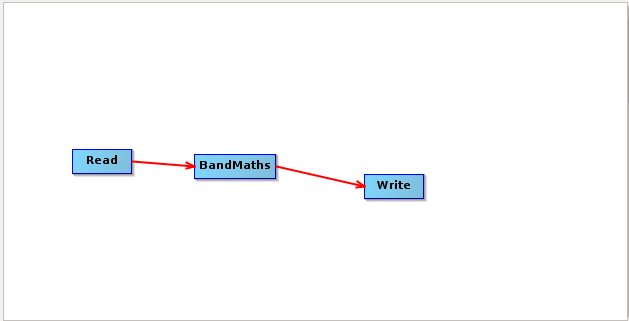# NDWI in S-2: error and problems

Dear all, I found several issues in calculating NDWI of a S-2 image, but any related topic in the forum.
In particular, I calculated NDWI in the graphical interface by using bandmath and it was OK.

Then I tried using bandmath in the graph builder, obtaining this strange result

May this be related to any parameter I haven’t set correctly (such as “Target band type”, “no-data value” and “band unit”)?

Finally I calculated NDWI in the interface by using the proper operator and an error message appeared

Please note that in all the cases the selected bands for calculating the index were B8A and B11.
I am particularly interested into calculate NDWI in the graph builder especially!
Thanks for helping!

Why you don’t calculate it in QGIS?

done in ENVI, just wanted to know why could not in SNAP!

hi gamato,

If you want to calculate indices using SENTINEL-2 data, there are a few different options

1. You can access EO browser . EO Browser provides indices (such as NDWI, NDVI, false colour, e.t.c) which have been pre-calculated and users and download them. So, you do not need to calculate the NDWI index from scratch. You can also use `custom scripts` and write the equations yourself as shown in the figure below

Figure1. NDWI calculation for Glasgow city

1. In case you want to compute your own indices using software packages (SNAP, QGIS), you can use EO explorer to download SENTINEL-2 images. The benefit of using EO explorer for downloading data is that you can select only the bands from Sentinel-2 you are interested in. Also, the bands have a tiff format. Hence, the size of the file is very small and manageable. You need to do the following:

-. select the sentinel-2 image over your AOI
-. load them in SNAP and stack them
-. calculate the index

Figure2. NDWI calculated in SNAP using data from EO explorer

1. If you want to download the whole product from copernicus schi-hub and calculate the index, here is the results.

Figure 3. NDWI index calculated from copernicous data

You can see the formula on the side for NDWI calculation. I do not really know the reason you got such strange results on your scene. I used graph builder and ti works for me
Bellow is the simple graph i have used.1 Like